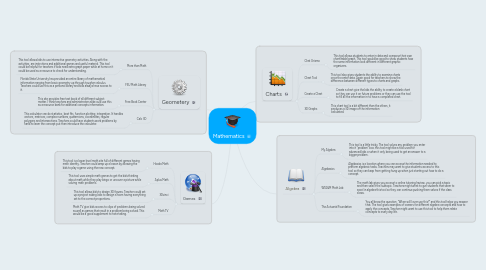# Mathematics

Get Started. It's FreeMathematics## 1. Charts

### 1.1. Chat Grizmo

1.1.1. This tool allows students to enter in data and compose their own chart/table/graph. This tool would be good to show students how the same information look different in different graphic organizers.

### 1.2. Chart Tool

1.2.1. This tool also gives students the ability to examine charts once the enter data. Again good for teachers to show the difference between different types to charts and graphs.

### 1.3. Create a Chart

1.3.1. Create a chart give the kids the ability to create a blank chart so they can use it on future problems or they can use the tool to fill all the information in to have a completed chart.

### 1.4. 3D Graphs

1.4.1. This chart tool is a bit different than the others, it produces a 3D image of the information calculated.

## 2. Algebra

### 2.1. My Algebra

2.1.1. This tool is a little tricky. The tool solves any problem you enter into it "problem" box. this tool might be a tool used for advanced kids or when it only being used to get an answer to a bigger problem.

### 2.2. Algebasics

2.2.1. Algebasics is a location where you can access the information needed to preform algebraic tasks. Teachers may want to give students access to this tool so they can keep from getting hung up when just starting out how to do a concept.

### 2.3. WTA&M Math Lab

2.3.1. The math lab gives you access to online tutoring/review. you can pick a topic and then select the subtopic. Teachers might what to gve students that seem to excel in algebra this tool so they can continue pushing them selves if the class slows.

### 2.4. The Actuarial Foundation

2.4.1. You all know the question, "When will I ever use this?" and this tool helps you answer that. The tool gives examples of careers for different algebra concepts and how to apply the concepts. Teacher might want to use this tool to help them relate concepts to every day life.

## 3. Geometery

### 3.1. More than Math

3.1.1. This tool allows kids to use interactive geometry activities. Along with the activities, are instrctions and additional games and useful material. This tool could be helpful for teachers if kids need extra graph paper while at home or it could be used as a resource to check for understanding.

### 3.2. FSU Math Library

3.2.1. Florida State University has provided an entire library of mathematical information ranging from basic geometry up through tougher calculus. Teachers could use this as a personal library and kids always have access to it.

### 3.3. Free Book Center

3.3.1. This site provides free text book of all different subject matter. I think teachers and administrators alike culd use this as a resource bank for addtional concepts information.

### 3.4. Calc 3D

3.4.1. This calculator can do statistics, best fits, function plotting, integration. It handles vectors, matrices, complex numbers, quaternions, coordinates, regular polygons and intersections. Teachers could have students work problems by hand to learn the concept put then introduce the calculator.

## 4. Games

### 4.1. Hooda Math

4.1.1. This tool is a lower level math site full of different games having math identity. Teacher could wrap up a lesson by allowing the kids to play a game using the new concept.

### 4.2. Aplus Math

4.2.1. This tool uses simple math games to get the kids thinking about math while they play bingo or uncover a picture while solving math problems.

### 4.3. 3Dvinci

4.3.1. This tool allows kids to design 3D figures. Teacher could set up a project asking kids to design a room having everything set to the correct proportions.

### 4.4. Math TV

4.4.1. Math TV give kids access to clips of problems being solved as well as games that result in a problem being solved. This would be a good supplement to note taking.